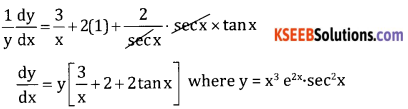# 2nd PUC Basic Maths Question Bank Chapter 18 Differential Calculus Ex 18.5

Students can Download Basic Maths Exercise 18.5 Questions and Answers, Notes Pdf, 2nd PUC Basic Maths Question Bank with Answers helps you to revise the complete Karnataka State Board Syllabus and score more marks in your examinations.

## Karnataka 2nd PUC Basic Maths Question Bank Chapter 18 Differential Calculus Ex 18.5

Part – A

2nd PUC Basic Maths Differential Calculus Ex 18.5 Two or Three Marks Questions and Answers

Question 1.
$$x^{\sqrt{x}}$$
Let y = $$x^{\sqrt{x}}$$ taking logm both sides
log y = $$\sqrt{x} \cdot \log x$$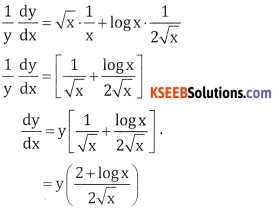Question 2.
xsin x
Let y = xsin x, taking logm both sudes
log y = sinx log x, Diff w.r.t x
$$\frac{1}{y} \cdot \frac{d y}{d x}$$ = sin x . $$\frac{1}{x}$$ + log x . cos x
$$\frac{d y}{d x}$$ = y[$$\frac{\sin x}{x}$$ + log x . cos x]Question 3.
(sin x)x.
Let y = (sin x)x, Taking logm
log y = x log sin x
$$\frac{1}{y} \frac{d y}{d x}$$ = x . $$\frac{1}{\sin x}$$ . cos x + logsin x.1
$$\frac{d y}{d x}$$ = y[x cot x + logsin x]

Question 4.
x5+log x
Let y = x5+log x, Taking logm both sides
log y = (5 + log x). log x,
Differentiate W.r.t x
$$\frac{1}{y} \cdot \frac{d y}{d x}$$ = (5 + log x) . $$\frac{1}{x}$$ + log(x) . $$\frac{1}{x}$$
$$\frac{d y}{d x}$$ = y $$\left[\frac{5+\log x+\log x}{x}\right]$$
= y$$\left[\frac{5+2 \log x}{x}\right]$$

Question 5.
x(sinx – cosx).
Let y = x(sinx – cosx) ; log y = (sin x – cos x) log x diff w.r.t x.
$$\frac{1}{y} \frac{d y}{d x}$$ = (sinx – cosx) . $$\frac{1}{x}$$ + log x (cos x + sin x);
$$\frac{d y}{d x}$$ = y [$$\frac{\sin x-\cos x}{x}$$ + log x.(cos x + sin x)]Part – B

2nd PUC Basic Maths Differential Calculus Ex 18.5 Five Marks Questions and Answers

Question 1.
xlogx + (log x)x
Let y = xlogx + (log x)x
y = u + v
$$\frac{d y}{d x}=\frac{d u}{d x}+\frac{d v}{d x}$$ …(1)
Where u = x and v = (log x)x
Taking logm both sides
log u = log x . log x log v = x log (log x)
Differentiate both w.r.t. x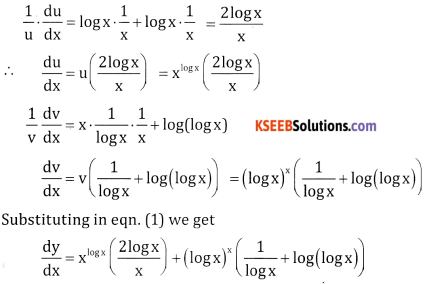Question 2.
x2 . ex2 . log x.
Let y = x2 . ex2 . log x. taking logm both sides
log y = log(x2 . ex2 . log x) log y = log (x2 . ex2 . log x) differentiate w.r.t x
log y = log x2 + log ex2 + log(log x)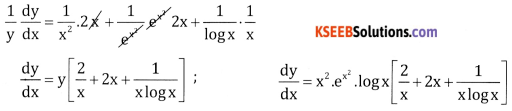Question 3.
(x + 1)2 (x + 2)3 (x + 3)4.
Let y = (x + 1)2 (x + 2)3 (x + 3)4, taking logm
log y = log(x + 1)2 + (x + 2)3 + (x + 3)4
log y = 2 log (x + 1) + 3 log (x + 2) + 4 log (x + 3)
Differentiate w.r.t x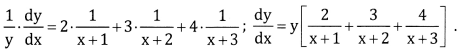Question 4.
x2x + xx2.
Let y = x2x + xx2
y = u + v
$$\frac{d y}{d x}=\frac{d u}{d x}+\frac{d v}{d x}$$ ….(1)
Where u = x2x and v = xx2
Taking logm both sides
log u = 2x logx logv = x2 log x
Differentiate both w.r.t x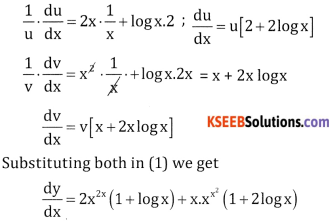Question 5.
$$=x^{\left(1+\frac{1}{x}\right)}+\left(1+\frac{1}{x}\right)^{x}$$.
Let y = $$=x^{\left(1+\frac{1}{x}\right)}+\left(1+\frac{1}{x}\right)^{x}$$ y = u + v $$\frac{d y}{d x}=\frac{d u}{d x}+\frac{d v}{d x}$$
Where u = $$x^{1+\frac{1}{x}}$$ v = $$\left(1+\frac{1}{x}\right)^{x}$$
Taking logm both sides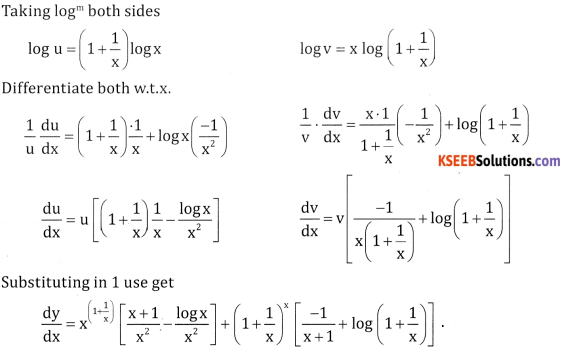Question 6.
$$\sqrt{\frac{\sqrt{(x-1)(x-2)}}{(x-3)(x-4)(x-5)}}$$
Let y = $$\sqrt{\frac{\sqrt{(x-1)(x-2)}}{(x-3)(x-4)(x-5)}}$$
Taking logm both sides
log y = $$\frac { 1 }{ 2 }$$ [log (x – 1) + log (x – 2) – log (x – 3) – log (x – 4) – log (x – 5)]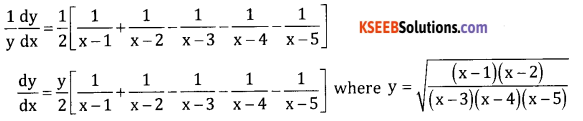Question 7.
x3 . e2x . sec2x.